maths > vector-algebra

Mathematical Representation of Vectors

what you'll learn...

Overview

Representation of Vectors

»  Specify amount or magnitude and direction

→  $3$$3$m east and $4$$4$m north

→  $3$$3$m east and $4$$4$m north-east

»  How were the quantities added?

→  $3$$3$m east + $4$$4$m north = $\sqrt{{3}^{2}+{4}^{2}}$$\sqrt{{3}^{2} + {4}^{2}}$ as per Pythagoras theorem

→  $3$$3$m east + $4$$4$m north-east : Making this easy to do is the objective of "vector algebra"

»  Position of an airplane $ai+bj+ck$$a i + b j + c k$

→  3Okm east (x-axis) = $30i$$30 i$

→  40km north (y-axis) = $40j$$40 j$

→  4km altitude (z-axis) = 4k

»  component form of a vector $ai+bj+ck$$a i + b j + c k$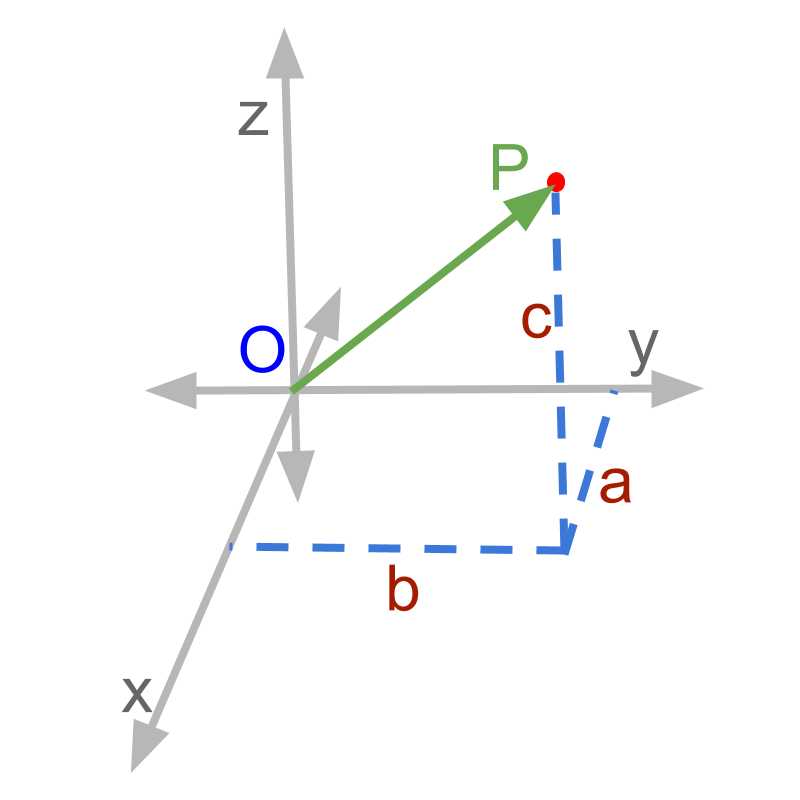→  $i$$i$,$j$$j$,$k$$k$ represent the direction along $x$$x$, $y$$y$, and $z$$z$ axes

→  $a$$a$, $b$$b$, $c$$c$ represent the amount or magnitude along the given directions

illustrative example

If someone is calculating the position of an airplane, a reasonable specification of the position is

$30$$30$km to the east, $40$$40$km north, and at $4$$4$km altitude above sea level

This specifies - North, East, and Altitude. With these three pieces of information, the position of the airplane is specified clearly.

We have arrived at representing vectors that have magnitude and direction.

The representation is
$30$$30$km East + $40$$40$km North + $4$$4$km Altitude

This can be generalized to a 3D space with three axes x-axis, y-axis, and z-axis. The figure represents x-axis, y-axis, and z-axis. A point 'p' is shown.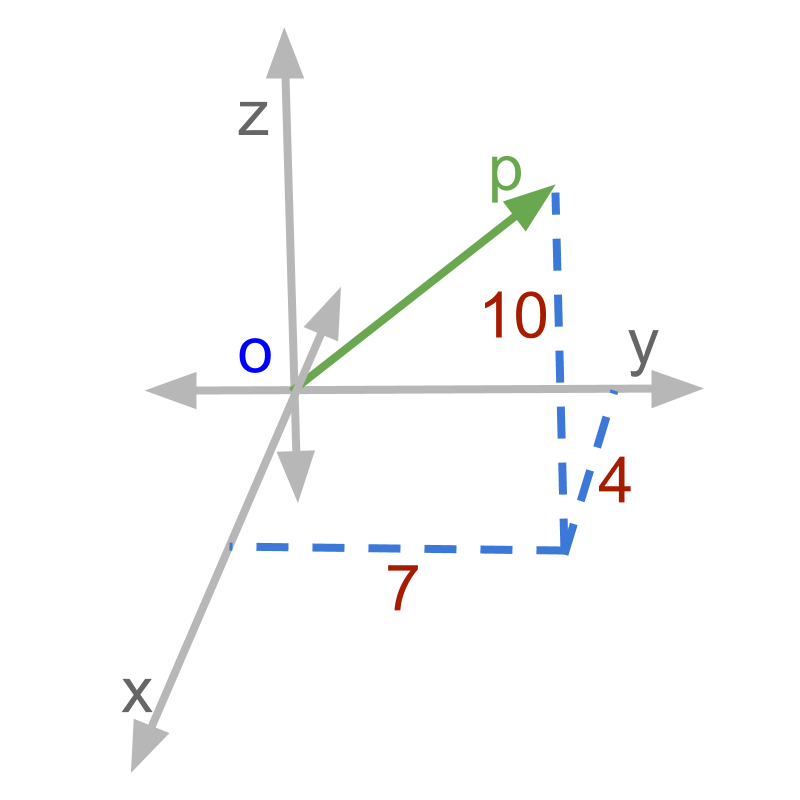The x, y, and z coordinates are given as $\left(x,y,z\right)$$\left(x , y , z\right)$. The point given in figure is '$\left(4,7,10\right)$$\left(4 , 7 , 10\right)$'.
The point is projected onto x-y plane. That is further projected onto x and y axes.

The airplane can be considered as the point 'p' in the three dimensional space. The representation is simplified as
$4i+7j+10k$$4 i + 7 j + 10 k$
or alternatively
$4\stackrel{^}{i}+7\stackrel{^}{j}+10\stackrel{^}{k}$$4 \hat{i} + 7 \hat{j} + 10 \hat{k}$
Where
$i$$i$ or $\stackrel{^}{i}$$\hat{i}$ represents the direction in $x$$x$-axis
$j$$j$ or $\stackrel{^}{j}$$\hat{j}$ represents the direction in $y$$y$-axis
$k$$k$ or $\stackrel{^}{k}$$\hat{k}$ represents the direction in $z$$z$-axis

Note: $\stackrel{^}{i}$$\hat{i}$ is pronounced as i-hat or i-cap.

A 3D vector quantity is represented with 3 components along the three directions of 3D coordinates.

Mathematical representation: A 3D vector quantity is represented in the form $ai+bj+ck$$a i + b j + c k$
or alternatively
$a\stackrel{^}{i}+b\stackrel{^}{j}+c\stackrel{^}{k}$$a \hat{i} + b \hat{j} + c \hat{k}$
Where i, j, k are the directions along x, y, and z axes
and a, b, c are the magnitude along the directions respectively.

examples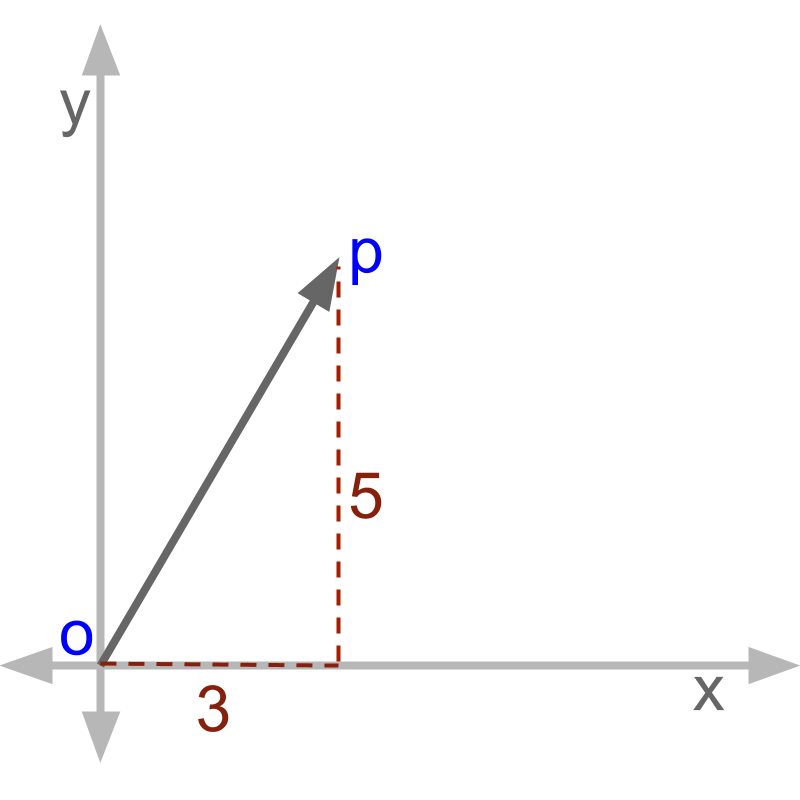Represent OP as a vector.The answer is '$3i+5j$$3 i + 5 j$'.
The x-axis component is represented with an $i$$i$ and y-axis component is represented with a $j$$j$.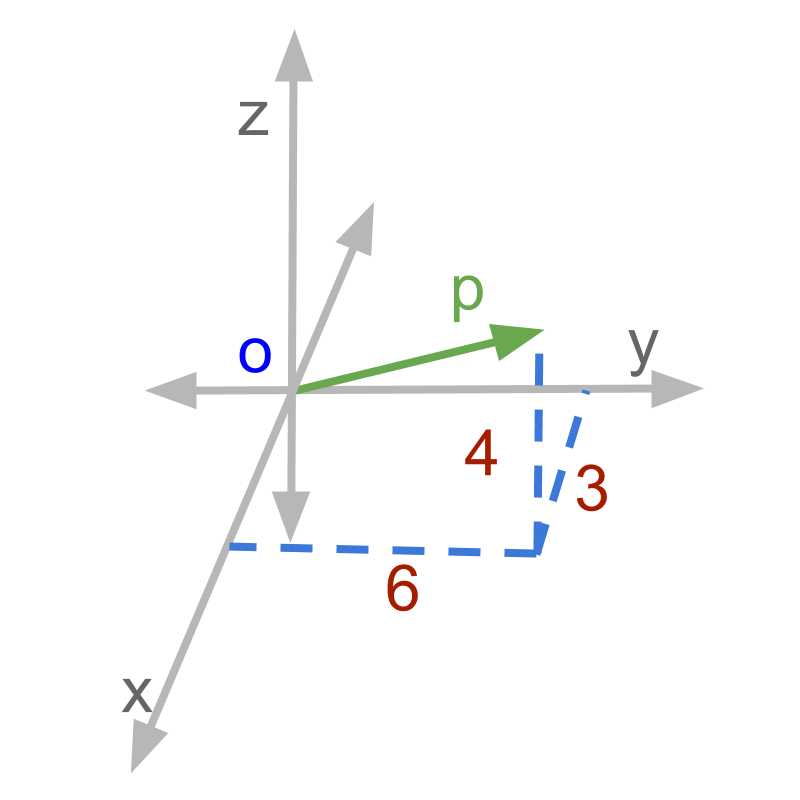What is the vector form of $\overline{OP}$$\overline{O P}$? The answer is '$3i+6j+4k$$3 i + 6 j + 4 k$'. Referring to the figure, the component along each of the axes
$3i$$3 i$: $3$$3$ along x axis
$6j$$6 j$: $6$$6$ along y axis
$4k$$4 k$: $4$$4$ along z axis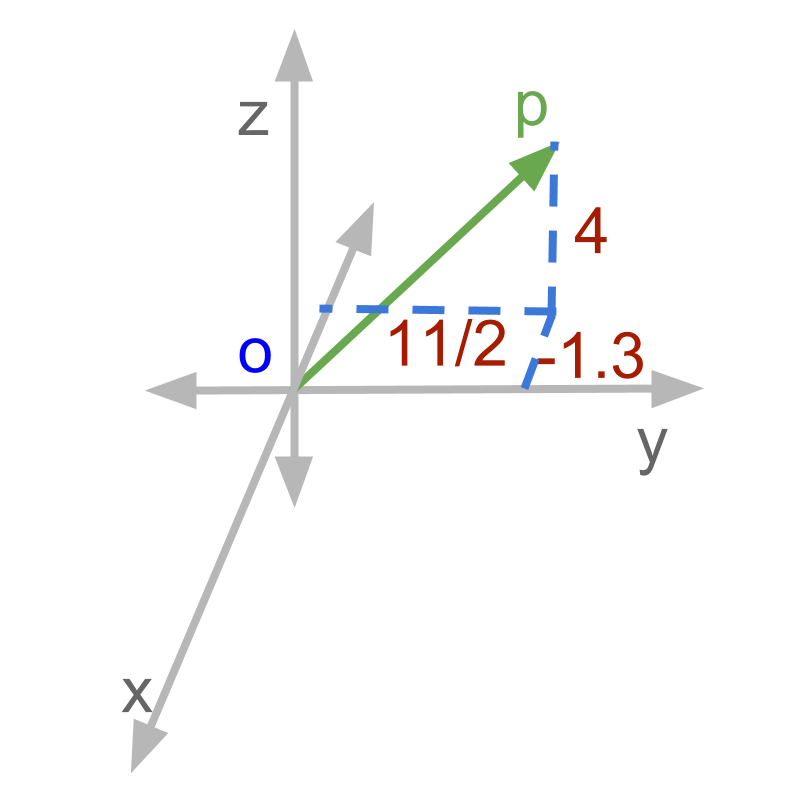What is the vector form of OP? The answer is '$-1.3i-\frac{11}{2}j+4k$$- 1.3 i - \frac{11}{2} j + 4 k$'.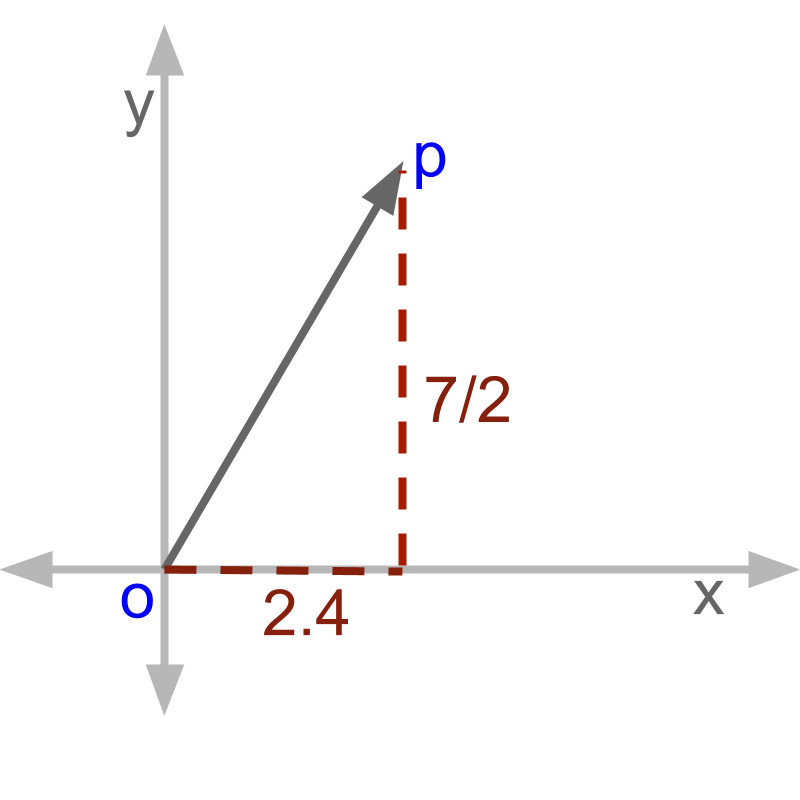What is the vector form of OP? $2.4i+\frac{7}{2}j$$2.4 i + \frac{7}{2} j$ or $2.4i+\frac{7}{2}j+0k$$2.4 i + \frac{7}{2} j + 0 k$ When a two dimensional vector is presented, the component along the third dimension is 0.

A point is located from the x, y, z-axes at distances $2$$2$, $-1.2$$- 1.2$, and $1.4$$1.4$ units respectively. What is the vector representation of the point?

The answer is '$2i-1.2j+1.4k$$2 i - 1.2 j + 1.4 k$'

What does a $\stackrel{\to }{OP}=-.5i+2.1j-.6k$ mean?
the point P is $-0.5$ unit away from origin along x-axis
the point P is $2.1$$2.1$ unit away from origin along y-axis
the point P is $-0.6$ unit away from origin along z-axis

summary

Mathematical representation: A 3D vector quantity is represented in the form $ai+bj+ck$$a i + b j + c k$
or alternatively
$a\stackrel{^}{i}+b\stackrel{^}{j}+c\stackrel{^}{k}$$a \hat{i} + b \hat{j} + c \hat{k}$
Where i, j, k are the directions along x, y, and z axes
and a, b, c are the magnitude along the directions respectively.Outline